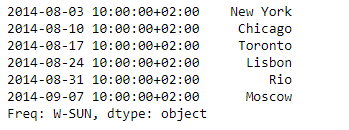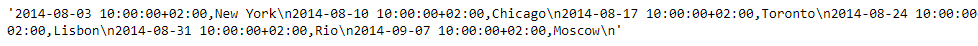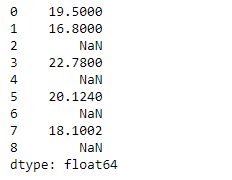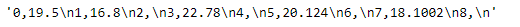Related Articles
Python | Pandas Series.to_csv()
• Last Updated : 24 Jun, 2020

Pandas series is a One-dimensional ndarray with axis labels. The labels need not be unique but must be a hashable type. The object supports both integer- and label-based indexing and provides a host of methods for performing operations involving the index.

Pandas` Series.to_csv()` function write the given series object to a comma-separated values (csv) file/format.

Syntax: Series.to_csv(*args, **kwargs)

Parameter :
path_or_buf : File path or object, if None is provided the result is returned as a string.
sep : String of length 1. Field delimiter for the output file.
na_rep : Missing data representation.
float_format : Format string for floating point numbers.
columns : Columns to write
header : If a list of strings is given it is assumed to be aliases for the column names.
index : Write row names (index).
index_label : Column label for index column(s) if desired. If None is given, and header and index are True, then the index names are used.
mode : Python write mode, default ‘w’.
encoding : A string representing the encoding to use in the output file.
compression : Compression mode among the following possible values: {‘infer’, ‘gzip’, ‘bz2’, ‘zip’, ‘xz’, None}.
quoting : Defaults to csv.QUOTE_MINIMAL.
quotechar : String of length 1. Character used to quote fields.

Returns : None or str

Example #1: Use `Series.to_csv()` function to convert the given series object to csv format.

 `# importing pandas as pd ` `import` `pandas as pd ` ` `  `# Creating the Series ` `sr ``=` `pd.Series([``'New York'``, ``'Chicago'``, ``'Toronto'``, ``'Lisbon'``, ``'Rio'``, ``'Moscow'``]) ` ` `  `# Create the Datetime Index ` `didx ``=` `pd.DatetimeIndex(start ``=``'2014-08-01 10:00'``, freq ``=``'W'``,  ` `                     ``periods ``=` `6``, tz ``=` `'Europe / Berlin'``)  ` ` `  `# set the index ` `sr.index ``=` `didx ` ` `  `# Print the series ` `print``(sr) `

Output :Now we will use `Series.to_csv()` function to convert the given Series object into a comma separated format.

 `# convert to comma-separated ` `sr.to_csv() `

Output :As we can see in the output, the `Series.to_csv()` function has converted the given Series object into a comma-separated format.

Example #2: Use `Series.to_csv()` function to convert the given series object to csv format.

 `# importing pandas as pd ` `import` `pandas as pd ` ` `  `# Creating the Series ` `sr ``=` `pd.Series([``19.5``, ``16.8``, ``None``, ``22.78``, ``None``, ``20.124``, ``None``, ``18.1002``, ``None``]) ` ` `  `# Print the series ` `print``(sr) `

Output :Now we will use `Series.to_csv()` function to convert the given Series object into a comma separated format.

 `# convert to comma-separated ` `sr.to_csv() `

Output :As we can see in the output, the `Series.to_csv()` function has converted the given Series object into a comma-separated format.

Attention geek! Strengthen your foundations with the Python Programming Foundation Course and learn the basics.

To begin with, your interview preparations Enhance your Data Structures concepts with the Python DS Course.

My Personal Notes arrow_drop_up
Recommended Articles
Page :